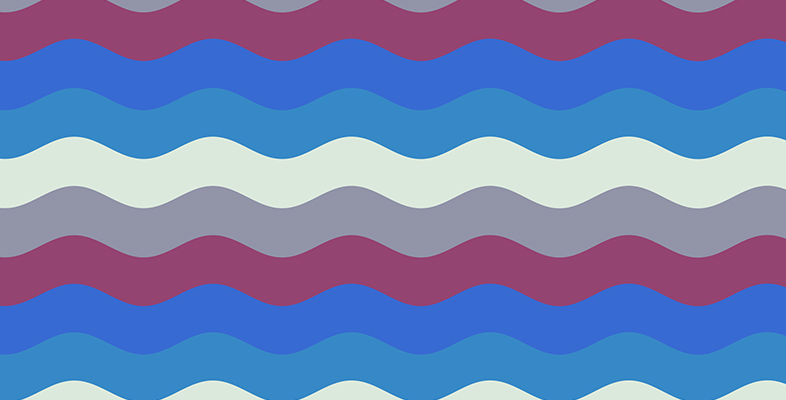Science, Maths & Technology

### Become an OU studentWhat are waves?

Start this free course now. Just create an account and sign in. Enrol and complete the course for a free statement of participation or digital badge if available.

# 3.1 Transverse waves

Figure 1 showed the expanding wave pattern from a stone splash, with the wave moving away from the initial disturbance. The oscillation, that is the up-and-down motion of the water, is at a right angle (90°) to the direction in which the wave propagates, so this is called a transverse wave.

With the stone splash, friction caused the oscillation to be damped and the wave faded. Imagine a system where there is no friction damping the oscillations. If there was a constant source of motion, a cross-section through the surface of the water as the wave moved past would appear as in Figure 2.Figure 2  The propagation of a transverse wave being generated on the left. The wave appears to move to the right. (Wave on a string, 2017)

In Figure 2 the dots mark fixed points on the surface and the dashed line the location of the undisturbed surface. Focus on locations on the wave where the distance above the undisturbed surface line is the greatest. Each of these places is a peak, and the distance from the undisturbed surface line to the peak is called the amplitude of the wave.

Each of the locations where the distance is the furthest below the undisturbed surface line is called a trough, and the distance from the undisturbed surface to a peak is the same as the distance to a trough. This means the distance from peak to trough is twice the amplitude.

• Do the locations of the peaks and troughs stay at the same point on the horizontal axis?

• No. The locations of the peaks and troughs move to the right.

We say that the wave is propagating to the right. Focusing on the peaks again, the distance from one peak to the next marks one complete cycle, and this distance is known as the wavelength. You can see in Figure 2 that the wavelength is constant. We do not have to choose one peak to the next peak to mark a wavelength – we could use any point on the wave, although of course from one peak to the next is very clear.

Observe what happens if some of the points are highlighted in green (Figure 3).Figure 3  A transverse wave with selected points highlighted in green.
• Do the green dots highlighted in the wave in Figure 3 travel to the right with the wave?

• No. The green dots only oscillate vertically about the undisturbed surface line. In fact, this is the case for all the dots.

While the points on the wave only move in a vertical direction, the wave propagates to the right. Transverse waves have particles moving at right angles to the direction of propagation.

The second type of wave is a longitudinal wave.